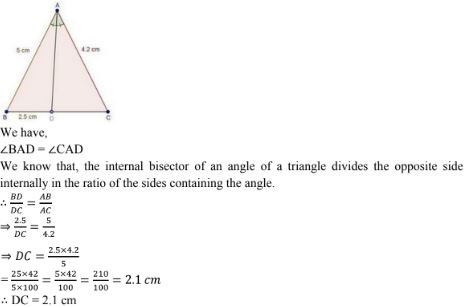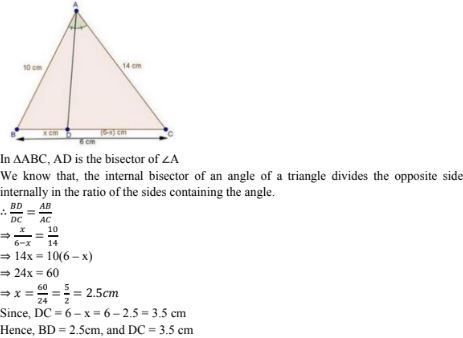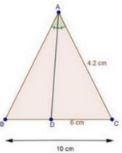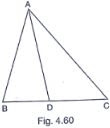# RD Sharma Solutions Chapter 4 Triangles Exercise 4.3 Class 10 Maths

 Chapter Name RD Sharma Chapter 4 Triangles Book Name RD Sharma Mathematics for Class 10 Other Exercises Exercise 4.1Exercise 4.2Exercise 4.4Exercise 4.5Exercise 4.6Exercise 4.7 Related Study NCERT Solutions for Class 10 Maths

### Exercise 4.3 Solutions

1. In a ΔABC, AD is the bisector of ∠A, meeting side BC at D.
(i) If BD = 2.5cm, AB = 5cm  and AC = 4.2cm, find DC.
(ii) If BD = 2cm, AB = 5cm and DC = 3cm, find AC.
(iii) If AB = 3.5 cm , AC = 4.2cm  and DC = 2.8 cm, find BD.
(iv) If AB = 10 cm, AC = 14cm and BC = 6cm, find BD and DC.
(v)  If  AC = 4.2cm, DC = 6 cm and 10cm, find AB
(vi) If AB = 5.6 cm, AC = 6cm and DC = 3 cm, find BC.
(vii) If AD = 5.6 cm, BC = 6cm and BD = 3.2cm, find AC.
(viii) If AB = 10 cm, Ac = 6 cm and BC = 12 cm, find BD and DC.

Solution

(i) If BD = 2.5cm, AB = 5cm  and AC = 4.2cm, find DC.(iii) If AB = 3.5 cm , AC = 4.2cm  and DC = 2.8 cm, find BD.

(iv) If AB = 10 cm, AC = 14cm and BC = 6cm, find BD and DC.(v)  If  AC = 4.2cm, DC = 6 cm and 10cm, find ABWe have,
BC = 10cm , DC = 6 cm and AC = 4.2cm
∴ BD = BC - DC = 10 - 6 = 4 cm
⇒ BD = 4cm
In ΔABC, AD is the bisector of ∠A.
We know that, the internal bisector of an angle of a triangle divides the opposite side internally in the ratio of the sides containing the angle.

(vi) If AB = 5.6 cm, AC = 6cm and DC = 3 cm, find BC.

(vii) If AD = 5.6 cm, BC = 6cm and BD = 3.2cm, find AC.

(viii) If AB = 10 cm, Ac = 6 cm and BC = 12 cm, find BD and DC.

2. In Fig 4.57, AE is the bisector of the exterior ∠CAD meeting BC produced in E. If AB = 10cm, AC = 6cm and BC = 12cm, find CE.
Solution

In ΔABC, AD is the bisector of ∠A.
We know that, the internal bisector of an angle of a triangle divides the opposite side internally in the ratio of the sides containing the angle.

3. In Fig. 4.58, ΔABC is a triangle such that AB/AC = BD/DC, ∠B = 70°, ∠C = 50° . Find ∠BAD .
Solution

We have, if a line through one vertex of a triangle divides the opposite side in the ratio of the other two sides, then the line bisects the angle at the vertex.

4. In ΔABC (fig., 4.59), if ∠1 = ∠2,  prove that AB/AC = BD/DC .
Solution

5. D, E and F are the points on sides BC, CA and AB respectively of ΔABC such that AD bisects ∠A, BE bisects ∠B and CF bisects ∠C. If AB = 5 cm, BC = 8 cm and CA = 4 cm, determine AP, CE and BD.
Solution

6. In fig., 4.60, check whether AD is the bisector of ∠A of ΔABC in each of the following :(i) AB = 5cm, AC = 10cm, BD = 1.5cm and CD = 3.5cm
(ii)  AB = 4cm, AC = 6 cm, BD = 1.6cm and CD = 2.4cm
(iii) AB = 8 cm, AC = 24 cm, BD = 6cm and BC = 24 cm
(iv) AB = 6cm, AC = 8cm , BD = 1.5cm and CD = 2cm.
(v) AB = 5cm, AC = 12cm, BD = 2.5cm and BC = 9 cm
Solution